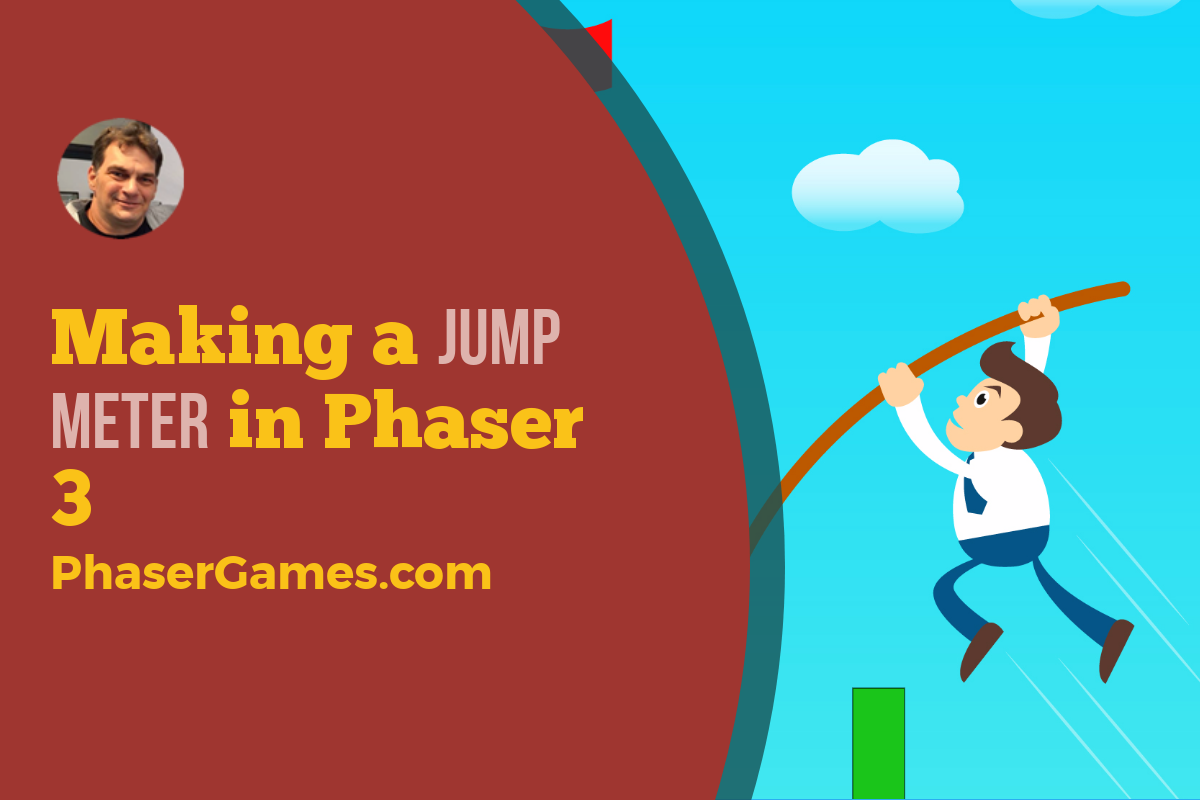# Make a Jump Meter in Phaser 3

In the last post, I showed how you can use gravity and velocity together to make a sprite jump. In this post, we will add a jump meter to the project to show the user just how much power they will use.

This project uses the Utility Template.

## Making the meter image

The first thing we will need is an image to make the meter line from. You can use a graphic object, but I find this does not work so well on older iPads.

I’m just going to use a simple green 25 x 25 square. Let’s start by loading the image in the preload function

``this.load.image("green","images/green.png");``

## Making the meter

For the meter, we just need to make an image game object. We will then grow and shrink that image based on how much power will be added to the jump.

Place this code at the end of the create function

``this.meter=this.add.image(0,0,"green");``

## Make an align Grid

I’m going to take advantage of the Utility Template’s alignGrid feature to place the meter near the ball. First, let’s build the alignGrid. Place this code just above where you added the meter code.

this.aGrid = new AlignGrid({
scene: this,
rows: 11,
cols: 11
});
this.aGrid.showNumbers();

## Placing the meter

Let’s place the meter in grid cell #100. You can change this to suit your own game later. Put this code just under where you create the meter

``this.aGrid.placeAtIndex(100, this.meter);``

## Showing Power

To grow the bar based on power we can change the scaleY property. Setting this to 2 will make the bar twice the height. Setting to 3, three times the height and so forth.

In the tick function add a line of code to set the scaleY property to equal the power

`````` tick() {
if (this.power < 5) {
this.power += .5;
this.meter.scaleY = this.power;
}
}``````

Setting the origin

If you run the code now, you’ll see that the bar grows from the center. To get the right effect we need it to grow from the bottom upwards. We can fix this by setting the origins of the bar to 1.

Just a note about origin settings. 0 means x,y, and scaling will start with the top, 0.5 refers to the center, and a 1 means the bottom. We can set an origin for both x and y.

Place this code just below the where you make the meter.

``this.meter.setOrigin(1, 1);``

The meter should now grow from the bottom upwards.

## Reset the meter

After the power is applied to the ball, we need to reset the meter. In the end jump function let’s reset the scaleY of the image. This will make the meter invisible until power is applied again.

``````endJump() {
this.timer.remove();
this.ball.setVelocityY(-this.power * 100);
this.power = 0;
this.meter.scaleY = 0;
}``````

We should also set the image’s scaleY when we create it as well. Add the line just after the origin code

`````` this.meter = this.add.image(0, 0, "green");
this.aGrid.placeAtIndex(100, this.meter);
this.meter.setOrigin(1, 1);
this.meter.scaleY = 0;``````

The last thing to do is to delete or comment out the showNumbers() code. Here is the final result

Here is the complete code below:

``````class SceneMain extends Phaser.Scene {
constructor() {
super('SceneMain');
}
}
create() {
this.power = 0;
//define our objects
//
//
//ball
this.ball = this.physics.add.sprite(this.sys.game.config.width / 2, 0, "ball");
this.ball.setGravityY(200);
//
//
//
//ground
//
//
let ground = this.physics.add.sprite(this.sys.game.config.width / 2, this.sys.game.config.height * .95, "block");
ground.displayWidth = this.sys.game.config.width * 1.1;
ground.setImmovable();
//
//
//
//set collider
//
//
this.input.on('pointerdown', this.startJump, this);
this.input.on('pointerup', this.endJump, this);
this.aGrid = new AlignGrid({
scene: this,
rows: 11,
cols: 11
});
//  this.aGrid.showNumbers();
this.aGrid.placeAtIndex(100, this.meter);
this.meter.setOrigin(1, 1);
this.meter.scaleY = 0;
}
endJump() {
this.timer.remove();
this.ball.setVelocityY(-this.power * 100);
this.power = 0;
this.meter.scaleY = 0;
}
startJump() {
delay: 100,
callback: this.tick,
callbackScope: this,
loop: true
});
}
tick() {
if (this.power < 5) {
this.power += .5;
this.meter.scaleY = this.power;
console.log(this.power);
}
}
update() {
//constant running loop
}
}``````